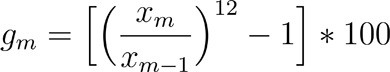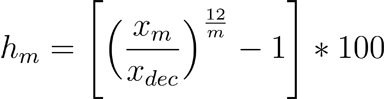Annualizing DataHow to annualize percent changes in quarterly and monthly data

The Economic Problem

Annualizing Data Facilitates Comparison of Growth Rates of Various Time Periods

Suppose Texas employment grew 0.92 percent in the first five months of a particular year. Then in June and July, employment advanced 0.15 percent and 0.22 percent, respectively. Would employment growth in June and July be above or below the pace set in the first five months of the year?

While this simple problem could probably be tackled in a few different ways, the most common one is a process called data annualization. In this method, growth rates are adjusted to reflect the amount a variable would have changed over a year’s time, had it continued to grow at the given rate. The result is a percent change that is easily comparable to other annualized data.

In this case, the 0.92 percent translates into an annualized 2.22 percent. The 0.15 becomes 1.81 percent (annualized), and the 0.22 figure becomes 2.67 percent (annualized). Thus, employment growth in June was below the rate established in the first five months, while the July figure was above it, in annualized terms. This kind of data adjustment is very common in economic analysis. It allows for quick comparison of percent changes, no matter the time period.

The Technical Solution

The formula for annualizing monthly data is straightforward:NOTE: For quarterly data, use 4 instead of 12.

where Xm and Xm – 1 are the values of the economic variable in months m and m –1, respectively (for example, m = February, then m – 1 = January), and gm is the annualized percent change.

For year-to-date calculations on monthly data, the formula is:NOTE: For quarterly data, use the fourth quarter instead of December, and q = 1, 2, 3, 4 instead of m = 1, 2, 3…12.

where XDec is the value of the economic variable in the December of a given year, m is the number of the month in question, Xm is the value of the economic variable in the mth month of the given year, and hm is the annualized year-to-m percent change.

Real-World Example

 Table 1 Month Employment (thousands) Monthly percent change (not annualized) Monthly percent change (annualized) December 9,452.5 n/a n/a January 9,465.2 .13 1.62 February 9,472.9 .08 0.98 March 9,498.3 .27 3.27 April 9,516.3 .19 2.30 May 9,539.5 .24 2.96 June 9,553.8 .15 1.81 July 9,574.8 .22 2.67 May/Dec n/a .92 2.22

On the July row, 0.22 is found by calculating the percent change between 9,553,800 (June) and 9,574,800 (July). The annualized figure of 2.67 is found by applying Equation 1: Divide 9,574,800 by 9,553,800, raise this quotient by 12, subtract 1, and multiply the whole thing by 100 (Calculation 1). This rate represents the amount employment would have increased for the year had it expanded at that monthly rate all 12 months. The calculation for the other months is the same.

In the last row, the 0.92 figure is found by calculating the simple percent change between 9,452,500 (December) and 9,539,500 (May). The annualized figure of 2.22 percent is found by applying Equation 2: Divide 9,539,500 by 9,452,500, raise this quotient by 2.4 (12/5), subtract 1, and multiply the whole thing by 100 (Calculation 2). This rate represents the amount employment would have increased for the year had it continued to expand at the pace set between January and May.

Summary

The annualizing methodology offers a simple way to compare the growth rates of economic variables presented across different periods. Analysts can regularly assess the monthly or quarterly performance of key economic indicators relative to their changes in recent years.

Note

Annualized rates of growth in monthly or quarterly data are generally only calculated for data that are not seasonal, or that have had the seasonality removed.

Glossary at a Glance
Annualize:
Adjusting a growth rate to reflect the amount a variable would have changed over a year's time had it continued to grow at the given rate.
DataBasics